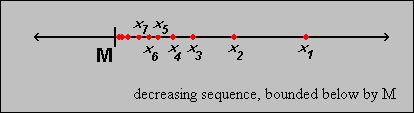#Interactive Real Analysis

Next | Previous | Glossary | Map

## 3.1. Sequences

### Proposition 3.1.9: Monotone Sequences

Ifis a monotone increasing sequence that is bounded above, then the sequence must converge (see picture).• Ifis a monotone decreasing sequence that is bounded below, then the sequence must converge (see picture).• ### Proof:

Let's look at the first statement, i.e. the sequence in monotone increasing. Take an> 0 and let c = sup(xk). Then c is finite, and given> 0, there exists at least one integer N such that xN > c -. Since the sequence is monotone increasing, we then have that
xk > c -for all k > N, or
| c - xk | <for all k > N. But that means, by definition, that the sequence converges to c.

The proof for the infimum is very similar, and is left as an exercise.Next | Previous | Glossary | Map